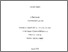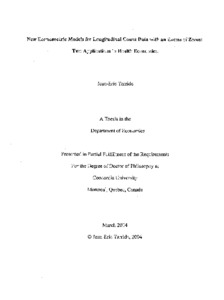Title:

# New econometric models for longitudinal count data with an excess of zeros : two applications in health economics

Tarride, Jean-Eric (2004) New econometric models for longitudinal count data with an excess of zeros : two applications in health economics. PhD thesis, Concordia University.Preview
Text (application/pdf)
NQ90405.pdf - Accepted Version
16MB

## Abstract

The purpose of this doctoral thesis is to provide new econometric models to analyze longitudinal count data characterized by a high proportion of zeros in the data. Previous econometric studies have dealt with many characteristics such as the discrete and longitudinal aspects of the dependent count variable or the presence of covariates and unobserved individual heterogeneity. However, none have taken into account the issues associated with an excess of zeros in a longitudinal framework. While it is well known in the univariate case that when an excess of zeros is significant, the mean has to be corrected to take into account this feature of the data, this issue has often been ignored in the longitudinal case. An excess of zeros in the data may lead to important modeling issues associated with the analysis of longitudinal count data. Two new econometric models are presented to address the six following characteristics: (1) count outcome, (2) a limited number of repeated measurements, (3) presence of covariates, (4) unobserved heterogeneity, (5) presence of correlation due to the repeated nature of the data and (6) an excess of zeros. The first model, a Quadrivariate Negative Binomial Hurdle model, was developed to analyze the number of doctor visits made by a panel of more than 4,000 German followed over 4 years. In the second example, a Quadrivariate Negative Binomial Zero-Inflated model was used to analyze an unpublished subset of a longitudinal clinical trial in which the treatments were very effective in reducing the number of occurrences of one variable collected over time in this trial. These two new models were nested to the Quadrivariate Negative Binomial model, allowing us to test for an excess of zeros. The main result is that the excess of zeros was significant in our two examples and assuming that only one process generates the data is incorrect. As such, the Multivariate Negative Binomial Hurdle and Zero-Inflated models are superior than standard Univariate Negative Binomial model, Quadrivariate Negative Binomial model and Generalized Estimating Equations model. These new models performed well in predicting the mean counts and the mean proportion of zeros in the data at each time period. This thesis demonstrated that caution should be taken in analyzing longitudinal count data in the presence of a high proportion of zeros in the data and correlation over time. Models ignoring these features may yield inconsistent estimates.

Divisions: Concordia University > Faculty of Arts and Science > Economics Thesis (PhD) Tarride, Jean-Eric xi, 196 leaves ; 29 cm. Concordia University Ph. D. Economics 2004 McIntosh, J 7866 Concordia University Library 18 Aug 2011 18:08 18 Jan 2018 17:31 http://clues.concordia.ca/search/c?SEARC...
All items in Spectrum are protected by copyright, with all rights reserved. The use of items is governed by Spectrum's terms of access.

Repository Staff Only: item control pageResearch related to the current document (at the CORE website)
Back to top